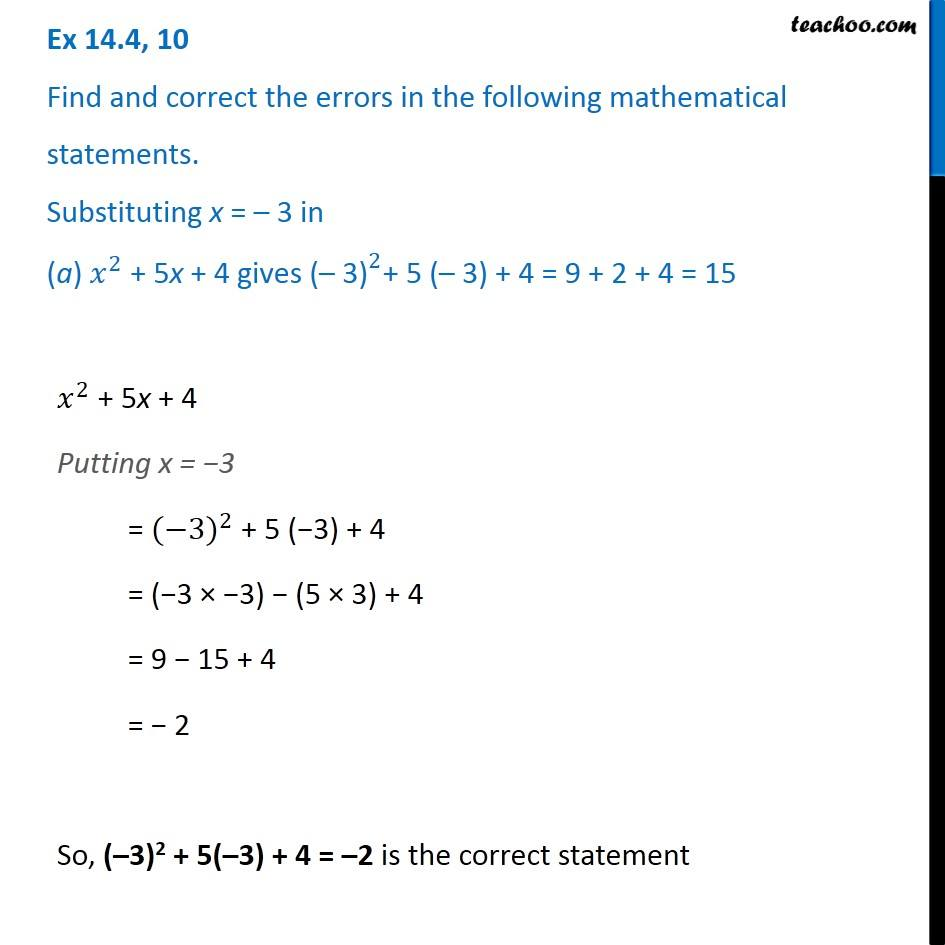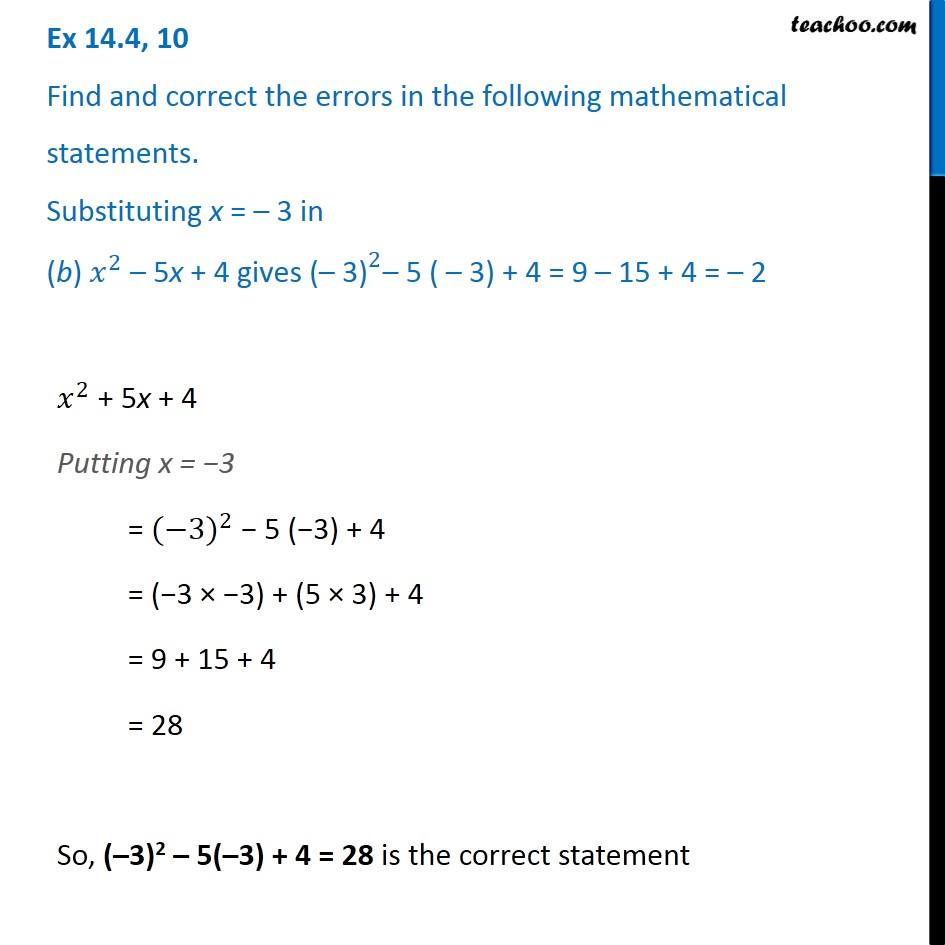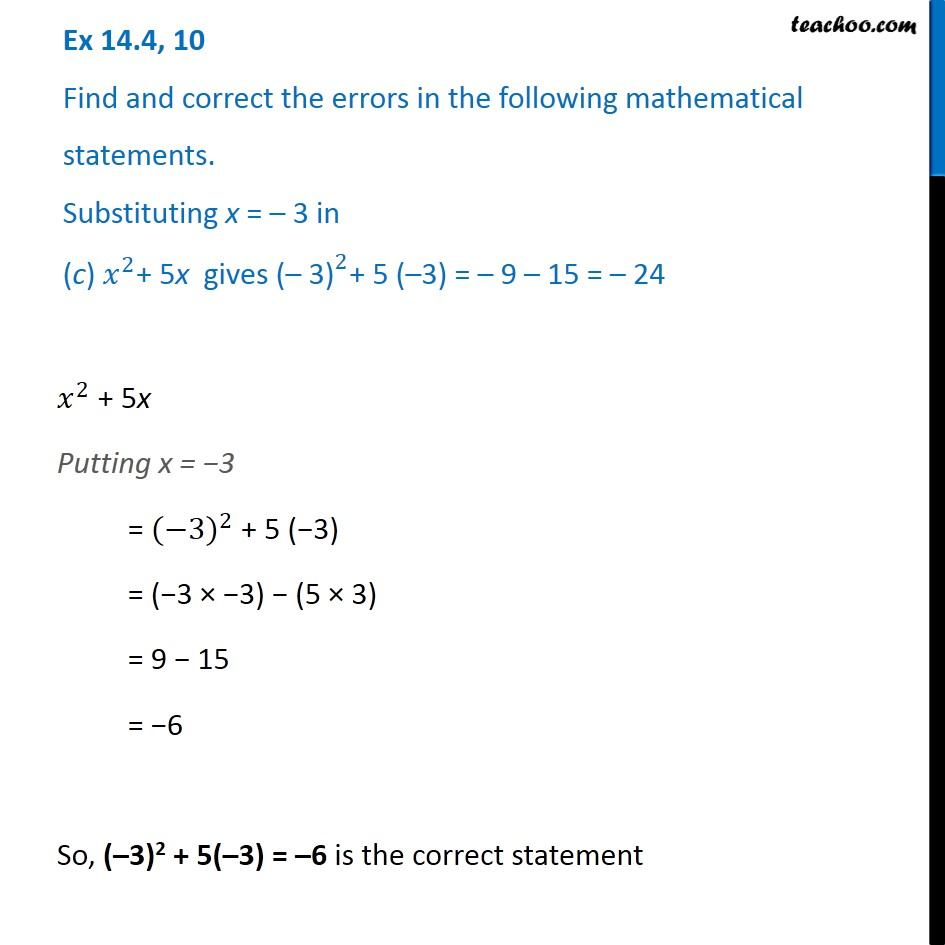Identify and correct errors in the sentences

Chapter 12 Class 8 Factorisation
Serial order wiseLearn in your speed, with individual attention - Teachoo Maths 1-on-1 Class

### Transcript

Question 10 Find and correct the errors in the following mathematical statements. Substituting x = – 3 in (a) 𝑥^2 + 5x + 4 gives 〖"(– 3)" 〗^2+ 5 (– 3) + 4 = 9 + 2 + 4 = 15 𝑥^2 + 5x + 4 Putting x = −3 = 〖(−3)〗^2 + 5 (−3) + 4 = (−3 × −3) − (5 × 3) + 4 = 9 − 15 + 4 = − 2 So, (–3)2 + 5(–3) + 4 = –2 is the correct statement Question 10 Find and correct the errors in the following mathematical statements. Substituting x = – 3 in (b) 𝑥^2 – 5x + 4 gives 〖"(– 3)" 〗^2– 5 ( – 3) + 4 = 9 – 15 + 4 = – 2 𝑥^2 + 5x + 4 Putting x = −3 = 〖(−3)〗^2 − 5 (−3) + 4 = (−3 × −3) + (5 × 3) + 4 = 9 + 15 + 4 = 28 So, (–3)2 – 5(–3) + 4 = 28 is the correct statement Question 10 Find and correct the errors in the following mathematical statements. Substituting x = – 3 in (c) 𝑥^2+ 5x gives 〖"(– 3)" 〗^2+ 5 (–3) = – 9 – 15 = – 24 𝑥^2 + 5x Putting x = −3 = 〖(−3)〗^2 + 5 (−3) = (−3 × −3) − (5 × 3) = 9 − 15 = −6 So, (–3)2 + 5(–3) = –6 is the correct statement